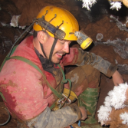Populating Point Variable in Modelbuilder

509
3
05-28-2020 03:55 PMNew Contributor II

I am trying to use Extract By Circle with the Center Point parameter being filled with the XCENTROID and YCENTROID fields gotten from a Zonal Geometry table. However, the Center Point parameter (or any Point variable for that matter) does not accept connectors (giving the error message "Only a variable can be connected to a process") and does not accept inline variable substitution such as %XCENTROID% (see attached screenshot with red box around entered text). What gives?

I could generate points, buffer them, and clip the raster instead, but Extract By Circle would greatly simplify my model, especially considering that I get the centroids from Zonal Statistics already.

Thanks!

3 RepliesbyMVP Esteemed Contributor

This is kind of fun once you figure out how it works!

Extract By Circle needs a object of type Point for that parameter, in the ExtractByCircle help they have a Python example that looks like this.

outExtCircle = ExtractByCircle("elevation", arcpy.Point(482838.823, 222128.982), 500, "INSIDE")‍‍‍

You need a string representation, because all variables in ModelBuilder are text strings, even when they represent other data types. These aren't always obvious (Point string rep would be  something  "100.0 00.0 NaN NaN" -- but most aren't that simple, think: Spatial Reference) so I like to construct them using the Calculate Value tool. You use this tool to create a Python string expression and assign its type to Point. Say X and Y are model variables you extracted from your table using Get Field Value. You set these as preconditions to the Calculate Value tool, then use:

Calculate Value expression: str(arcpy.Point(%X%, %Y%))

Calculate Value data type: Point

The output is a text representation of a Point, which  you assign as Point type using the data type parameter. If you do this, you can connect the point value output to Extract By Circle!New Contributor II

Thanks for this! It lets me connect the Point variable to the Extract By Circle tool, but when I run the model (from the toolbox) it fails with "ERROR 000859: The required parameter Center point is empty, or is not the type of Point." It seems that Modelbuilder cannot validate the Extract By Circle tool until Calculate Value has run, I'm guessing because Calculate Value does not have a default output like '1 1' that Extract By Circle would recognize as a point. Is there any way to validate the model with these tools?

modelbuilder‌ geoprocessing tool problembyMVP Frequent Contributor

To compliment Curtis Price‌'s answer this is why you to need to read the help file and understand what it is showing you, especially the syntax section of each tool. If you look at the data type of centre_point it is a POINT geometry. This would have helped you realise that you can't connect a number variable and would have hopefully lead you down the path of creating a geometry object. Your idea of a buffer centroid approach would have worked but would have added extra steps into the model whilst Curtis's slick solution introduces only 1 tool.

So if models are not doing what you expect have a look at the syntax section of the tool.